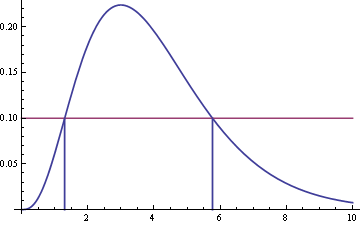# Finding the shortest interval with given mass

Here’s an elegant little theorem applied in statistics but useful more generally. Suppose you have a density function f(x) with one hump. Suppose a and b are two points on opposite sides of the hump with f(a) = f(b). Then [a, b] is the shortest interval with its mass. That is, any other interval of length ba will have less mass than the interval [a, b]. (Here the “mass” of an interval is just the integral of f(x) over that interval.)

Suppose we want to find the shortest interval that has a given mass k. Start by imagining a horizontal line sitting on top of the graph of f(x).Now lower this horizontal line so that it intersects the graph in two places.Draw vertical lines down from these two points of intersection to find their x-coordinates.In this example, the two x-coordinates are about 1.30 and 5.77. So the interval [1.30, 5.77] is the shortest interval with its mass. In other words, no other interval of length 4.47 can contain more mass than this interval does.

We can find the shortest interval of mass k by lowering this horizontal line until the interval it defines has mass k. The lower the horizontal line, the greater the mass. So for any given mass less than the total mass f(x) assigns, there is a unique height of the horizontal line that defines an interval with that mass.

This procedure could be used to find the shortest confidence interval or the shortest Bayesian credible interval. In that case the “mass” is probability, and the task is to find the shortest interval containing a specified probability. The theorem says that the shortest confidence interval or credible interval has equal probability density at each end of the interval.

A proof of this theorem is given in Statistical Inference, chapter 9. Technically, f(x) must be unimodal and positive with finite integral. A homework exercise in the same chapter outlines a simpler proof using the additional assumption that f(x) is continuous.

Related post: What is a confidence interval?